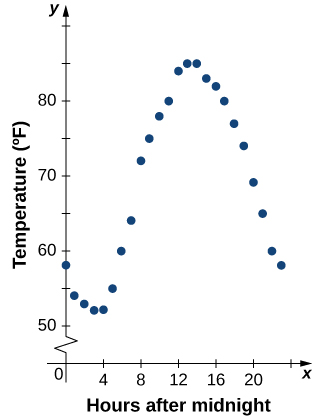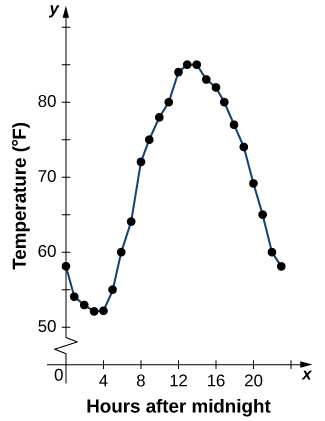# 1.1 Review of functions  (Page 4/28)

 Page 4 / 28

Find the domain and range for $f\left(x\right)=\sqrt{4-2x}+5.$

Domain = $\left\{x|x\le 2\right\},$ range = $\left\{y|y\ge 5\right\}$

## Representing functions

Typically, a function is represented using one or more of the following tools:

• A table
• A graph
• A formula

We can identify a function in each form, but we can also use them together. For instance, we can plot on a graph the values from a table or create a table from a formula.

## Tables

Functions described using a table of values    arise frequently in real-world applications. Consider the following simple example. We can describe temperature on a given day as a function of time of day. Suppose we record the temperature every hour for a 24-hour period starting at midnight. We let our input variable $x$ be the time after midnight, measured in hours, and the output variable $y$ be the temperature $x$ hours after midnight, measured in degrees Fahrenheit. We record our data in [link] .

Temperature as a function of time of day
Hours after Midnight Temperature $\left(\text{°}F\right)$ Hours after Midnight Temperature $\left(\text{°}F\right)$
0 58 12 84
1 54 13 85
2 53 14 85
3 52 15 83
4 52 16 82
5 55 17 80
6 60 18 77
7 64 19 74
8 72 20 69
9 75 21 65
10 78 22 60
11 80 23 58

We can see from the table that temperature is a function of time, and the temperature decreases, then increases, and then decreases again. However, we cannot get a clear picture of the behavior of the function without graphing it.

## Graphs

Given a function $f$ described by a table, we can provide a visual picture of the function in the form of a graph. Graphing the temperatures listed in [link] can give us a better idea of their fluctuation throughout the day. [link] shows the plot of the temperature function.The graph of the data from [link] shows temperature as a function of time.

From the points plotted on the graph in [link] , we can visualize the general shape of the graph. It is often useful to connect the dots in the graph, which represent the data from the table. In this example, although we cannot make any definitive conclusion regarding what the temperature was at any time for which the temperature was not recorded, given the number of data points collected and the pattern in these points, it is reasonable to suspect that the temperatures at other times followed a similar pattern, as we can see in [link] .Connecting the dots in [link] shows the general pattern of the data.

## Algebraic formulas

Sometimes we are not given the values of a function in table form, rather we are given the values in an explicit formula. Formulas arise in many applications. For example, the area of a circle of radius $r$ is given by the formula $A\left(r\right)=\pi {r}^{2}.$ When an object is thrown upward from the ground with an initial velocity ${v}_{0}$ ft/s, its height above the ground from the time it is thrown until it hits the ground is given by the formula $s\left(t\right)=-16{t}^{2}+{v}_{0}t.$ When $P$ dollars are invested in an account at an annual interest rate $r$ compounded continuously, the amount of money after $t$ years is given by the formula $A\left(t\right)=P{e}^{rt}.$ Algebraic formulas are important tools to calculate function values. Often we also represent these functions visually in graph form.

Given an algebraic formula for a function $f,$ the graph of $f$ is the set of points $\left(x,f\left(x\right)\right),$ where $x$ is in the domain of $f$ and $f\left(x\right)$ is in the range. To graph a function given by a formula, it is helpful to begin by using the formula to create a table of inputs and outputs. If the domain of $f$ consists of an infinite number of values, we cannot list all of them, but because listing some of the inputs and outputs can be very useful, it is often a good way to begin.

#### Questions & Answers

find the domain and range of f(x)= 4x-7/x²-6x+8
Nick Reply
find the range of f(x)=(x+1)(x+4)
Jane Reply
-1, -4
Marcia
That's domain. The range is [-9/4,+infinity)
Jacob
If you're using calculus to find the range, you have to find the extrema through the first derivative test and then substitute the x-value for the extrema back into the original equation.
Jacob
Good morning,,, how are you
Harrieta Reply
d/dx{1/y - lny + X^3.Y^5}
mogomotsi Reply
How to identify domain and range
Umar Reply
hello
Akpevwe
He,,
Harrieta
hi
Dr
hello
velocity
I only talk to girls
Dr
women are smart then guys
Dr
Smarter
Adri
sorry
Dr
hi adri ana
Dr
:(
Shun
was up
Dr
hello
Adarsh
is it chatting app?.. I do not see any calculus here. lol
Adarsh
Find the arc length of the graph of f(x) = In (sinx) on the interval [Π/4, Π/2].
mukul Reply
Sand falling freely from a lorry form a conical shape whose height is always equal to one-third the radius of the base. a. How fast is the volume increasing when the radius of the base is (1m) and increasing at the rate of 1/4cm/sec Pls help me solve
ade
show that lim f(x) + lim g(x)=m+l
BARNABAS Reply
list the basic elementary differentials
Chio Reply
Differentiation and integration
Okikiola Reply
yes
Damien
proper definition of derivative
Syed Reply
the maximum rate of change of one variable with respect to another variable
Amdad
terms of an AP is 1/v and the vth term is 1/u show that the sum of uv terms is 1/2(uv+1)
Inembo Reply
what is calculus?
BISWAJIT Reply
calculus is math that studies the change in math, such as the rate and distance,
Tamarcus
what are the topics in calculus
Augustine
what is limit of a function?
Geoffrey Reply
what is x and how x=9.1 take?
Pravin Reply
what is f(x)
Inembo Reply
the function at x
Marc
also known as the y value so I could say y=2x or f(x)= 2x same thing just using functional notation your next question is what is dependent and independent variables. I am Dyslexic but know math and which is which confuses me. but one can vary the x value while y depends on which x you use. also
Marc
up domain and range
Marc
enjoy your work and good luck
Marc
I actually wanted to ask another questions on sets if u dont mind please?
Inembo
I have so many questions on set and I really love dis app I never believed u would reply
Inembo
Hmm go ahead and ask you got me curious too much conversation here
Adri
am sorry for disturbing I really want to know math that's why *I want to know the meaning of those symbols in sets* e.g n,U,A', etc pls I want to know it and how to solve its problems
Inembo
and how can i solve a question like dis *in a group of 40 students, 32 offer maths and 24 offer physics and 4 offer neither maths nor physics , how many offer both maths and physics*
Inembo
next questions what do dy mean by (A' n B^c)^c'
Inembo
The sets help you to define the function. The function is like a magic box where you put inside stuff(numbers or sets) and you get out the stuff but in different shapes (forms).
Adri
I dont understand what you wanna say by (A' n B^c)^c'
Adri
(A' n B (rise to the power of c)) all rise to the power of c
Inembo
Aaaahh
Adri
Ok so the set is formed by vectors and not numbers
Adri
A vector of length n
Adri
But you can make a set out of matrixes as well
Adri
I I don't even understand sets I wat to know d meaning of all d symbolsnon sets
Inembo
Wait what's your math level?
Adri
High-school?
Adri
yes
Inembo
am having big problem understanding sets more than other math topics
Inembo
So f:R->R means that the function takes real numbers and provides real numer. For ex. If f(x) =2x this means if you give to your function a real number like 2,it gives you also a real number 2times2=4
Adri
pls answer this question *in a group of 40 students, 32 offer maths and 24 offer physics and 4 offer neither maths nor physics , how many offer both maths and physics*
Inembo
If you have f:R^n->R^n you give to your function a vector of length n like (a1,a2,...an) where all a1,.. an are reals and gives you also a vector of length n... I don't know if i answering your question. Otherwise on YouTube you havr many videos where they explain it in a simple way
Adri
I would say 24
Adri
Offer both
Adri
Sorry 20
Adri
Actually you have 40 - 4 =36 who offer maths or physics or both.
Adri
I know its 20 but how to prove it
Inembo
You have 32+24=56who offer courses
Adri
56-36=20 who give both courses... I would say that
Adri
solution: In a question involving sets and Venn diagram, the sum of the members of set A + set B - the joint members of both set A and B + the members that are not in sets A or B = the total members of the set. In symbolic form n(A U B) = n(A) + n (B) - n (A and B) + n (A U B)'.
Mckenzie
In the case of sets A and B use the letters m and p to represent the sets and we have: n (M U P) = 40; n (M) = 24; n (P) = 32; n (M and P) = unknown; n (M U P)' = 4
Mckenzie
Now substitute the numerical values for the symbolic representation 40 = 24 + 32 - n(M and P) + 4 Now solve for the unknown using algebra: 40 = 24 + 32+ 4 - n(M and P) 40 = 60 - n(M and P) Add n(M and P), as well, subtract 40 from both sides of the equation to find the answer.
Mckenzie
40 - 40 + n(M and P) = 60 - 40 - n(M and P) + n(M and P) Solution: n(M and P) = 20
Mckenzie
thanks
Inembo
Simpler form: Add the sums of set M, set P and the complement of the union of sets M and P then subtract the number of students from the total.
Mckenzie
n(M and P) = (32 + 24 + 4) - 40 = 60 - 40 = 20
Mckenzie

### Read also:

#### Get the best Calculus volume 1 course in your pocket!

Source:  OpenStax, Calculus volume 1. OpenStax CNX. Feb 05, 2016 Download for free at http://cnx.org/content/col11964/1.2
Google Play and the Google Play logo are trademarks of Google Inc.

Notification Switch

Would you like to follow the 'Calculus volume 1' conversation and receive update notifications?By Lakeima RobertsBy Anonymous UserBy RhodesBy Abishek DevarajBy Edward Biton